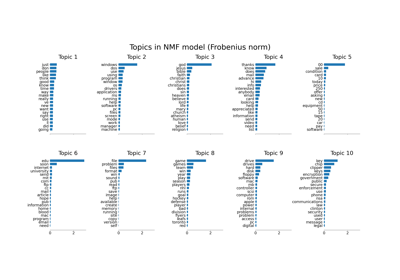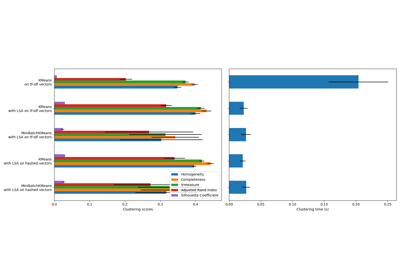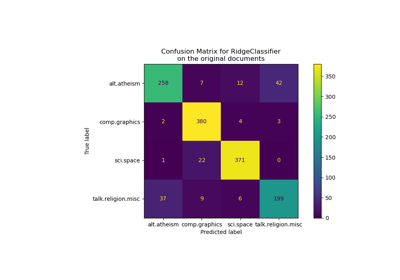# sklearn.feature_extraction.text.TfidfVectorizer¶

class sklearn.feature_extraction.text.TfidfVectorizer(*, input='content', encoding='utf-8', decode_error='strict', strip_accents=None, lowercase=True, preprocessor=None, tokenizer=None, analyzer='word', stop_words=None, token_pattern='(?u)\b\w\w+\b', ngram_range=(1, 1), max_df=1.0, min_df=1, max_features=None, vocabulary=None, binary=False, dtype=<class 'numpy.float64'>, norm='l2', use_idf=True, smooth_idf=True, sublinear_tf=False)[source]

Convert a collection of raw documents to a matrix of TF-IDF features.

Equivalent to CountVectorizer followed by TfidfTransformer.

Read more in the User Guide.

Parameters
input{‘filename’, ‘file’, ‘content’}, default=’content’

If ‘filename’, the sequence passed as an argument to fit is expected to be a list of filenames that need reading to fetch the raw content to analyze.

If ‘file’, the sequence items must have a ‘read’ method (file-like object) that is called to fetch the bytes in memory.

Otherwise the input is expected to be a sequence of items that can be of type string or byte.

encodingstr, default=’utf-8’

If bytes or files are given to analyze, this encoding is used to decode.

decode_error{‘strict’, ‘ignore’, ‘replace’}, default=’strict’

Instruction on what to do if a byte sequence is given to analyze that contains characters not of the given encoding. By default, it is ‘strict’, meaning that a UnicodeDecodeError will be raised. Other values are ‘ignore’ and ‘replace’.

strip_accents{‘ascii’, ‘unicode’}, default=None

Remove accents and perform other character normalization during the preprocessing step. ‘ascii’ is a fast method that only works on characters that have an direct ASCII mapping. ‘unicode’ is a slightly slower method that works on any characters. None (default) does nothing.

Both ‘ascii’ and ‘unicode’ use NFKD normalization from unicodedata.normalize.

lowercasebool, default=True

Convert all characters to lowercase before tokenizing.

preprocessorcallable, default=None

Override the preprocessing (string transformation) stage while preserving the tokenizing and n-grams generation steps. Only applies if analyzer is not callable.

tokenizercallable, default=None

Override the string tokenization step while preserving the preprocessing and n-grams generation steps. Only applies if analyzer == 'word'.

analyzer{‘word’, ‘char’, ‘char_wb’} or callable, default=’word’

Whether the feature should be made of word or character n-grams. Option ‘char_wb’ creates character n-grams only from text inside word boundaries; n-grams at the edges of words are padded with space.

If a callable is passed it is used to extract the sequence of features out of the raw, unprocessed input.

Changed in version 0.21.

Since v0.21, if input is filename or file, the data is first read from the file and then passed to the given callable analyzer.

stop_words{‘english’}, list, default=None

If a string, it is passed to _check_stop_list and the appropriate stop list is returned. ‘english’ is currently the only supported string value. There are several known issues with ‘english’ and you should consider an alternative (see Using stop words).

If a list, that list is assumed to contain stop words, all of which will be removed from the resulting tokens. Only applies if analyzer == 'word'.

If None, no stop words will be used. max_df can be set to a value in the range [0.7, 1.0) to automatically detect and filter stop words based on intra corpus document frequency of terms.

token_patternstr

Regular expression denoting what constitutes a “token”, only used if analyzer == 'word'. The default regexp selects tokens of 2 or more alphanumeric characters (punctuation is completely ignored and always treated as a token separator).

ngram_rangetuple (min_n, max_n), default=(1, 1)

The lower and upper boundary of the range of n-values for different n-grams to be extracted. All values of n such that min_n <= n <= max_n will be used. For example an ngram_range of (1, 1) means only unigrams, (1, 2) means unigrams and bigrams, and (2, 2) means only bigrams. Only applies if analyzer is not callable.

max_dffloat or int, default=1.0

When building the vocabulary ignore terms that have a document frequency strictly higher than the given threshold (corpus-specific stop words). If float in range [0.0, 1.0], the parameter represents a proportion of documents, integer absolute counts. This parameter is ignored if vocabulary is not None.

min_dffloat or int, default=1

When building the vocabulary ignore terms that have a document frequency strictly lower than the given threshold. This value is also called cut-off in the literature. If float in range of [0.0, 1.0], the parameter represents a proportion of documents, integer absolute counts. This parameter is ignored if vocabulary is not None.

max_featuresint, default=None

If not None, build a vocabulary that only consider the top max_features ordered by term frequency across the corpus.

This parameter is ignored if vocabulary is not None.

vocabularyMapping or iterable, default=None

Either a Mapping (e.g., a dict) where keys are terms and values are indices in the feature matrix, or an iterable over terms. If not given, a vocabulary is determined from the input documents.

binarybool, default=False

If True, all non-zero term counts are set to 1. This does not mean outputs will have only 0/1 values, only that the tf term in tf-idf is binary. (Set idf and normalization to False to get 0/1 outputs).

dtypedtype, default=float64

Type of the matrix returned by fit_transform() or transform().

norm{‘l1’, ‘l2’}, default=’l2’

Each output row will have unit norm, either: * ‘l2’: Sum of squares of vector elements is 1. The cosine similarity between two vectors is their dot product when l2 norm has been applied. * ‘l1’: Sum of absolute values of vector elements is 1. See preprocessing.normalize.

use_idfbool, default=True

Enable inverse-document-frequency reweighting.

smooth_idfbool, default=True

Smooth idf weights by adding one to document frequencies, as if an extra document was seen containing every term in the collection exactly once. Prevents zero divisions.

sublinear_tfbool, default=False

Apply sublinear tf scaling, i.e. replace tf with 1 + log(tf).

Attributes
vocabulary_dict

A mapping of terms to feature indices.

fixed_vocabulary_: bool

True if a fixed vocabulary of term to indices mapping is provided by the user

idf_array of shape (n_features,)

The inverse document frequency (IDF) vector; only defined if use_idf is True.

stop_words_set

Terms that were ignored because they either:

• occurred in too many documents (max_df)

• occurred in too few documents (min_df)

• were cut off by feature selection (max_features).

This is only available if no vocabulary was given.

CountVectorizer

Transforms text into a sparse matrix of n-gram counts.

TfidfTransformer

Performs the TF-IDF transformation from a provided matrix of counts.

Notes

The stop_words_ attribute can get large and increase the model size when pickling. This attribute is provided only for introspection and can be safely removed using delattr or set to None before pickling.

Examples

>>> from sklearn.feature_extraction.text import TfidfVectorizer
>>> corpus = [
...     'This is the first document.',
...     'This document is the second document.',
...     'And this is the third one.',
...     'Is this the first document?',
... ]
>>> vectorizer = TfidfVectorizer()
>>> X = vectorizer.fit_transform(corpus)
>>> print(vectorizer.get_feature_names())
['and', 'document', 'first', 'is', 'one', 'second', 'the', 'third', 'this']
>>> print(X.shape)
(4, 9)


Methods

 Return a callable that handles preprocessing, tokenization and n-grams generation. Return a function to preprocess the text before tokenization. Return a function that splits a string into a sequence of tokens. decode(doc) Decode the input into a string of unicode symbols. fit(raw_documents[, y]) Learn vocabulary and idf from training set. fit_transform(raw_documents[, y]) Learn vocabulary and idf, return document-term matrix. Array mapping from feature integer indices to feature name. get_params([deep]) Get parameters for this estimator. Build or fetch the effective stop words list. Return terms per document with nonzero entries in X. set_params(**params) Set the parameters of this estimator. transform(raw_documents[, copy]) Transform documents to document-term matrix.
__init__(*, input='content', encoding='utf-8', decode_error='strict', strip_accents=None, lowercase=True, preprocessor=None, tokenizer=None, analyzer='word', stop_words=None, token_pattern='(?u)\\b\\w\\w+\\b', ngram_range=(1, 1), max_df=1.0, min_df=1, max_features=None, vocabulary=None, binary=False, dtype=<class 'numpy.float64'>, norm='l2', use_idf=True, smooth_idf=True, sublinear_tf=False)[source]

Initialize self. See help(type(self)) for accurate signature.

build_analyzer()[source]

Return a callable that handles preprocessing, tokenization and n-grams generation.

Returns
analyzer: callable

A function to handle preprocessing, tokenization and n-grams generation.

build_preprocessor()[source]

Return a function to preprocess the text before tokenization.

Returns
preprocessor: callable

A function to preprocess the text before tokenization.

build_tokenizer()[source]

Return a function that splits a string into a sequence of tokens.

Returns
tokenizer: callable

A function to split a string into a sequence of tokens.

decode(doc)[source]

Decode the input into a string of unicode symbols.

The decoding strategy depends on the vectorizer parameters.

Parameters
docstr

The string to decode.

Returns
doc: str

A string of unicode symbols.

fit(raw_documents, y=None)[source]

Learn vocabulary and idf from training set.

Parameters
raw_documentsiterable

An iterable which yields either str, unicode or file objects.

yNone

This parameter is not needed to compute tfidf.

Returns
selfobject

Fitted vectorizer.

fit_transform(raw_documents, y=None)[source]

Learn vocabulary and idf, return document-term matrix.

This is equivalent to fit followed by transform, but more efficiently implemented.

Parameters
raw_documentsiterable

An iterable which yields either str, unicode or file objects.

yNone

This parameter is ignored.

Returns
Xsparse matrix of (n_samples, n_features)

Tf-idf-weighted document-term matrix.

get_feature_names()[source]

Array mapping from feature integer indices to feature name.

Returns
feature_nameslist

A list of feature names.

get_params(deep=True)[source]

Get parameters for this estimator.

Parameters
deepbool, default=True

If True, will return the parameters for this estimator and contained subobjects that are estimators.

Returns
paramsmapping of string to any

Parameter names mapped to their values.

get_stop_words()[source]

Build or fetch the effective stop words list.

Returns
stop_words: list or None

A list of stop words.

inverse_transform(X)[source]

Return terms per document with nonzero entries in X.

Parameters
X{array-like, sparse matrix} of shape (n_samples, n_features)

Document-term matrix.

Returns
X_invlist of arrays of shape (n_samples,)

List of arrays of terms.

set_params(**params)[source]

Set the parameters of this estimator.

The method works on simple estimators as well as on nested objects (such as pipelines). The latter have parameters of the form <component>__<parameter> so that it’s possible to update each component of a nested object.

Parameters
**paramsdict

Estimator parameters.

Returns
selfobject

Estimator instance.

transform(raw_documents, copy='deprecated')[source]

Transform documents to document-term matrix.

Uses the vocabulary and document frequencies (df) learned by fit (or fit_transform).

Parameters
raw_documentsiterable

An iterable which yields either str, unicode or file objects.

copybool, default=True

Whether to copy X and operate on the copy or perform in-place operations.

Deprecated since version 0.22: The copy parameter is unused and was deprecated in version 0.22 and will be removed in 0.24. This parameter will be ignored.

Returns
Xsparse matrix of (n_samples, n_features)

Tf-idf-weighted document-term matrix.

## Examples using sklearn.feature_extraction.text.TfidfVectorizer¶